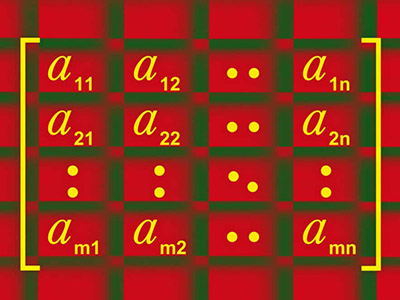ΓραμματείαSecretariat: 2410 684574 | ΦοιτητικάStudents: 2410 684387 g-ds@uth.gr
Select Page

# Linear Algebra

Υ306

3

#### Hours/Week - ECTS

4 – 5### Learning Outcomes

The main goal of the course is to fully understand the basic concepts of Linear Algebra which are necessary for the continuation of studies. Part of the aim of the course is to acquire specific technical knowledge (such as how I can solve a linear system, how I can calculate its eigenvalues, …) which in themselves will be completely useless.

Specifically, students will be able to

• Demonstrate their ability to understand and use the basic ideas of linear algebra, including the concepts of linear independence, linear transformations, bases and dimensions of vector spaces, eigenvalues, eigenvectors and quadratic.
• Compose clear and accurate evidence, using the concepts of the course.

### Indicative Module Content

• Determine if a system of equations has a solution and find its general solution.
• Analyze a table into a product of simpler tables.
• Solve systems of the form Ax = b, where A is an array/matrix mxn and x is a vector of Rn
• Determine whether the columns of a given table are linearly dependent or not.
• Understand what is the linear transformation defined by x → Ax.
• Recognize different categories of special tables.
• Calculate the determinant of a given table.
• Identify the four fundamental subspaces of a given table and find their bases and conclude the existence and uniqueness of the solution.
• Define a subspace from a vector space.
• Change the coordinates of a vector from the base to a standard base.
• Calculate the solution of least squares problems.
• Define the characteristic polynomial of a given array.
• Calculate the eigenvalues ​​and eigenvectors of a table.